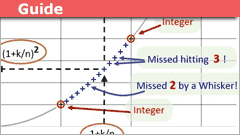# Irrationality for Dummies - Comments

• Insights
swamp-thing submitted a new PF Insights post

Irrationality for DummiesContinue reading the Original PF Insights Post.

•trilobite, Jimster41 and Greg Bernhardt

PeroK
Homework Helper
Gold Member
2021 Award
If you take a proper rational ##q = \frac{m}{n}## where ##m, n## have no common factors, then ##q^2 = \frac{m^2}{n^2}## is clearly a proper rational. Where would the common factors of ##m^2, n^2## come from? (To be rigorous, appeal to the fundamental theorem of arithmetic and unique prime factorisations).

In any case, proper rationals square to proper rationals, never to whole numbers. Hence, only whole numbers and irrationals can square to whole numbers.

Isn't that it in a nutshell?

Last edited:
•ShayanJ
Haven't checked the maths thoroughly but I really like your style of writing.

If you take a proper rational ##q = frac{m}{n}## where ##m, n## have no common factors, then ##q^2 = frac{m^2}{n^2}## is clearly a proper rational. Where would the common factors of ##m^2, n^2## come from? (To be rigorous, appeal to the fundamental theorem of arithmetic and unique prime factorisations).

In any case, proper rationals square to proper rationals, never to whole numbers. Hence, only whole numbers and irrationals can square to whole numbers.

Isn't that it in a nutshell?
PeroK : proper rationals square to proper rationals

Undeniably! :)

Haven't checked the maths thoroughly but I really like your style of writing.

Thank you, JorisL.

micromass
Staff Emeritus
Homework Helper
In your Insight you posted some kind of "walk" according to ##(1+ k/n)^2##. You said (and proved) that while doing this walk, you'll never land on an integer. Here's a question though: do you get arbitrarily close to an integer? For example, do you get closer than ##0.000001## to some integer?

In your Insight you posted some kind of "walk" according to ##(1+ k/n)^2##. You said (and proved) that while doing this walk, you'll never land on an integer. Here's a question though: do you get arbitrarily close to an integer? For example, do you get closer than ##0.000001## to some integer?
Hi micromass,
Interesting question. Here is a graph showing the result of walking from 1 to 2, 2 to 3 and so on - all superposed together. Some points are so close to integers that you can't tell by eye. But when you check the numbers, the nearest approach to an integer is 1/(n^2).

In this example, n=27 and the nearest approach to integers is 0.001372

Last edited by a moderator:
•Jimster41
If you take a proper rational ##q = \frac{m}{n}## where ##m, n## have no common factors, then ##q^2 = \frac{m^2}{n^2}## is clearly a proper rational. Where would the common factors of ##m^2, n^2## come from? (To be rigorous, appeal to the fundamental theorem of arithmetic and unique prime factorisations).

In any case, proper rationals square to proper rationals, never to whole numbers. Hence, only whole numbers and irrationals can square to whole numbers.

Isn't that it in a nutshell?

This thread has reminded me that my head is still spinning from trying to read M. Schroeder's book on Chaos Fractals and Power Laws - something that left me feeling deeply confused by the idea of division with remainders - among other things.

I don't know what the most general categorical type of "a number" would be, but assuming that there are things in nature that exactly correspond to that category what in the world can it mean for there to be two such things ostensibly made from the same space-time and matter, that have "No common factors"?

The plot in post #7 (like many in Schroeder's book) is just too eerily natural looking (or has the rhythmic arrhythmia so common in natural structures) to not beg (at least for me) such a bothersome question.

In other words why isn't any apple related to any other apple in an integer way? Or do we think that it is? Down at the level of the ultimate least common denominator - whatever bits of quanta that might be - are there some bits that are somehow "prime" relative to other bits?

Last edited:
PAllen
dummies need no help with irrationality.

Ancient Greek mathematicians freaked out when they discovered that the square root of 2 is not rational. Like Swamp Thing, they were not dummies and realized that the existence of irrational numbers is a fact that is remarkable, deep, and a little scary.

•Logical Dog
Ancient Greek mathematicians freaked out when they discovered that the square root of 2 is not rational. Like Swamp Thing, they were not dummies and realized that the existence of irrational numbers is a fact that is remarkable, deep, and a little scary.

Was someone really murdered over it? Aristarchus? or a similar sounding name (apologies for the historical inaccuracy)

Svein
Approximating irrationality: Using Newtons formula for the square root:

Assume that you have an approximation for the square root of 2: pn/qn. Then you can find a better approximation pn+1/qn+1 where pn+1 = pn2+2⋅qn2 and qn+1 = 2⋅pn⋅qn.

Here the "2" in the nominator is the number we are taking the square root of, and the "2" in the deniminator is just a 2.

You can start with almost any sensible number. A good start is q0 = 1 and p0 either 1 or the number you are taking the square root of.

Starting with 1 and 1:
1/1
3/ 2
17/12
577/408
665857/470832
...

Last edited:
Was someone really murdered over it? Aristarchus? or a similar sounding name (apologies for the historical inaccuracy)
Hippasus, according to Wikipedia, which says the story may be just legend.